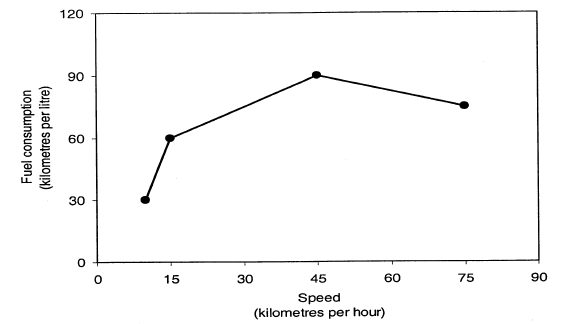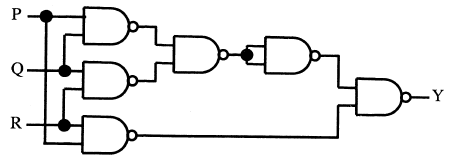Click to Answer GATE Question of the Day

### GATE Question of the Day - General Aptitude and Digital Question

After seeing a good response from many GATE aspirants to my yesterday post GATE Question of the Day -  General Aptitude Question . Today i am going to post a GATE General Aptitude Question and a question from Digital Electronics. General Aptitude is common to all branches and Digital Electronics is common to CSE, ECE, EEE and Instrumentation.

## The fuel consumed by a motorbike during a journey while traveling at different speed is given in the below graph## From the given data, We can conclude that the fuel consumed per kilometer was least during the lap

A)P  B)Q  C)R  D)S

## The output Y of the below circuit is always "1" when?A) Two are more of the inputs P, Q, R are "0"
B) Two are more of the inputs P, Q, R are "1"
C) Any odd number of the inputs P, Q, R are "0"
D) Any odd number of the inputs P, Q, R are "1"

Fuel consumed per kilometer was least during the lap will be 'Q' since it will cover 90km/hr.so the correct answer is option B for 1st question.

1) For P laps bike has to travel 15KM at the mileage of 30KM/L so fuel consumption per KM is 0.0333 Litre (333 ml)
2)For Q laps bike has to travel 75 KM at the mileage of 90KM/L so fuel consumption per KM is 0.0111 litre
(111 ml)
3)For R laps the bike has to travel 40KM at the mileage of 80KM/L(approximately) so fuel consumption per KM is 0.0125 litre (124 ml)
4)for S laps it has to travel 10KM at the mileage of 30KM/L so fuel consumption per KM is 0.0333 litre (333 ml)
Based on the above results the fuel consumption per KM during the Q Lap is less

For second question answer is B

Solution for the second question is

Y=(((PQ)'(QR)')(PQ)') = (((P'+Q')(Q'+R')(P'+Q'))=((P'Q'+P'R'+Q'+Q'R')(P'+Q'))

Y=(P'Q'+P'R'+P'Q'R'+Q'+Q'R')= PQ+QR+PR

So that two or more inputs are 1 for Y is always 1

Vamshi said...

first one is A,second one is B

Unknown said...

Ans to first que is A or D (i hav a doubt.... pls Vamshi reddy explain me how u got A as ans... plsss), Ans to secon que is B.

Kanchan Gupta said...

I think First one is A.

Kanchan Gupta said...

Vamshi said...

miss rani... just take the fuel consumed per kilometre according to that graph and find out that for the distance given in the table...

Unknown said...

Than k u very much

Vamshi said...

can you explain me how did you get the answer for the first one in detail plz...

Venkatesan Muniappan said...

For the first one I calculated the fuel consumption as follows
1) For P laps bike has to travel 15KM at the mileage of 60KM/L so fuel consumption is 250ml

2)For Q laps bike has to travel 75 KM at the mileage of 90KM/L so fuel consumption is 833ml

3)For R laps the bike has to travel 40KM at the mileage of 80KM/L(approximately) so fuel consumption is 500ml

4)for S laps it has to travel 10KM at the mileage of 30KM/L so fuel consumption is 333ml

Based on the above results the answer is P Laps

Notify me if I have mistake

Examsavvy said...

Vamshi said...

To calculate the fuel consumption for that problem,there is no need to consider the table ... then why it was given.... just to confuse us.... right..

Examsavvy said...

ya you are correct vamshi

Venkatesan Muniappan said...

@ Sundar Mano
fuel consumption is 33.33ml, 11.11ml,12.4ml and 33.33ml respectively for P,Q,R and S laps

One small request Please post at least one question per day to make this interesting

Examsavvy said...

Hi venkat, thanks for correcting my mistakes.

I can post questions daily, but i need some one to help me to organize this. can you help me?Anonymous said...

1st is C